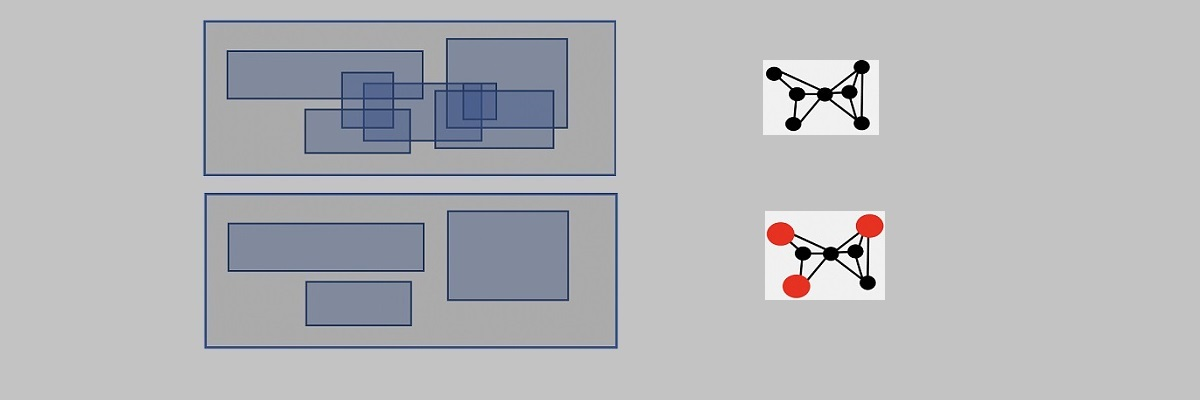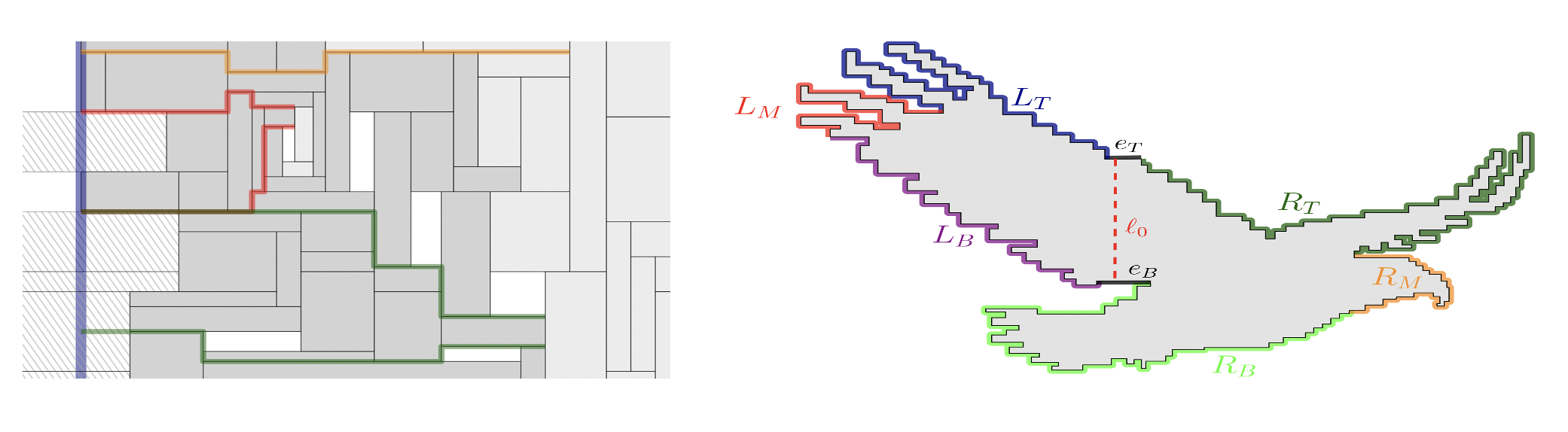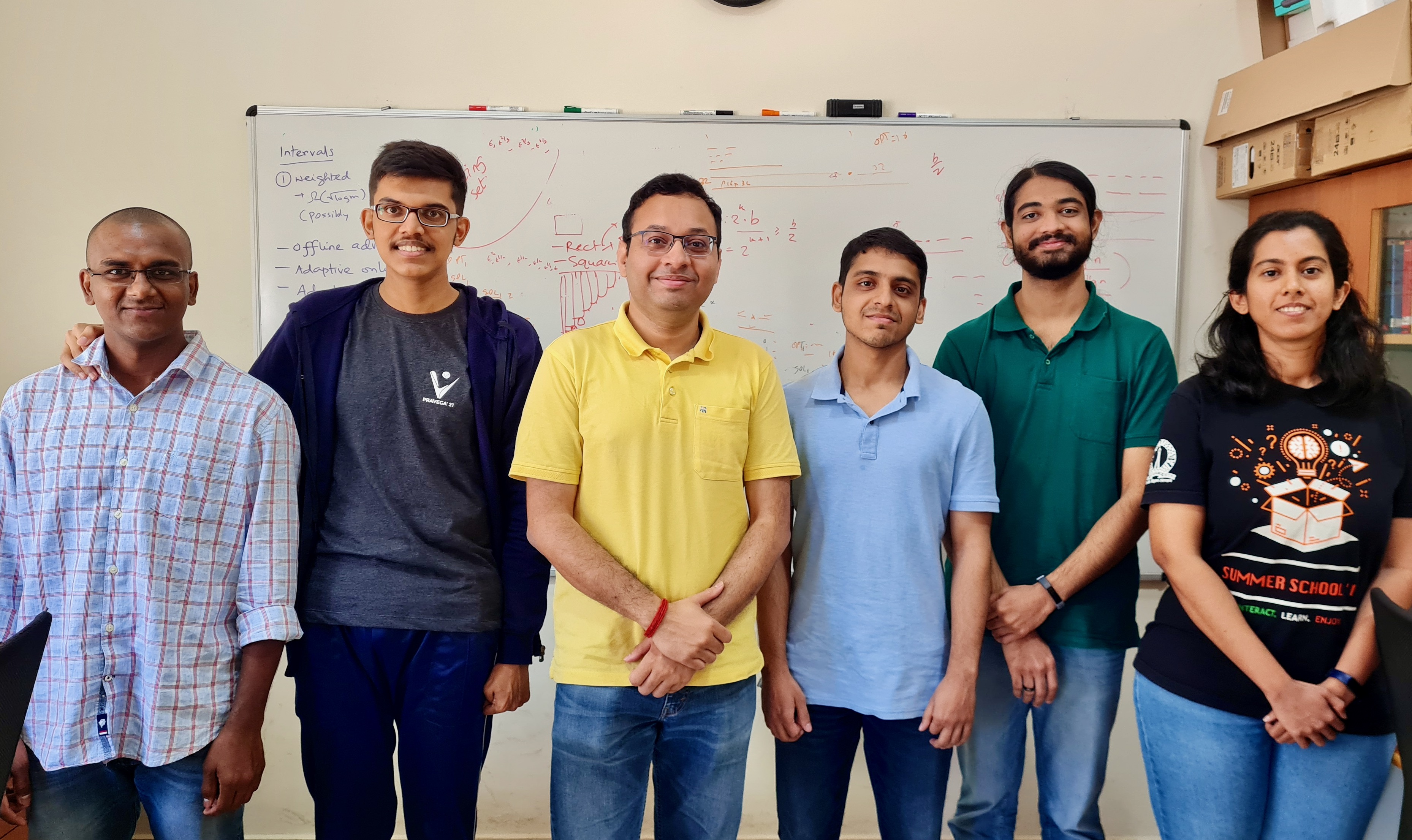# Breakthrough result in computational geometryMaximum Independent Set of Rectangles (MISR) is a fundamental problem in computational geometry, approximation algorithms, and combinatorial optimization. In this problem, given a set of (possibly overlapping) rectangles on a plane one needs to find the maximum number of non-overlapping set of rectangles. MISR finds numerous applications in practice, e.g., in map labeling, data mining, and resource allocation. It also has rich connections with many important problems in theoretical computer science and discrete geometry.However, it is intractable (NP-hard) to efficiently (in time polynomial in the input size) find the optimal solution for this problem. So researchers are trying to find good approximate solutions that are close to optimal.

It is conjectured that one can efficiently find solutions arbitrarily close to the optimal solution. However, it was a long-standing open problem to even obtain a solution within a constant factor of the optimal solution. Joe Mitchell in his seminal result  resolved this by showing an algorithm that finds a solution within one-tenth of the optimal solution.

Dr. Arindam Khan, his intern Madhusudhan Reddy, and their international collaborators from Chile, Germany, and Poland, made progress on this notoriously hard problem and gave an efficient algorithm that finds a solution that is at least one-third of the optimal solution. The result  is going to appear in ACM-SIAM Symposium on Discrete Algorithms (SODA), 2022 – one of the top conferences in Computer Science. Following the work, they have further improved the result and showed an efficient algorithm  that finds a solution (almost) half of the optimal solution. The work develops new mathematical techniques and extends the present techniques to their limits.

References:

 Waldo Gálvez, Arindam Khan, Mathieu Mari, Tobias Mömke, Madhusudhan Reddy Pittu, Andreas Wiese: A 3-Approximation Algorithm for Maximum Independent Set of Rectangles. To appear in ACM-SIAM Symposium on Discrete Algorithms (SODA), 2022.

 Waldo Gálvez, Arindam Khan, Mathieu Mari, Tobias Mömke, Madhusudhan Reddy Pittu, Andreas Wiese: A (2+\epsilon)-Approximation Algorithm for Maximum Independent Set of Rectangles. CoRR abs/2106.00623 (2021)
https://arxiv.org/pdf/2106.00623.pdf

 Joseph S. B. Mitchell: Approximating Maximum Independent Set for Rectangles in the Plane. To appear in IEEE Symposium on Foundations of Computer Science (FOCS), 2021.